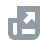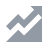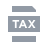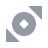شراء العملات الرقمية
الدفع بواسطة
الأسواق
تداول
المشتقات
أرباح
NFT
خدمات المؤسسات
الموجز
Cancel

# الأسئلة الأكثر شيوعاًوظائف الحسابالبرنامج التعليمي للمستخدمBinance Fan Tokenأرباح Binanceإيداع\سحب العملات الرقميةشراء العملات الرقمية (النقد الإلزامي\P2P)التداول الفوري والاقتراضي وتداول الصفقات الخارجيةمشتقات العملات الرقميةبوتات التداولالموارد الماليةAPIالحمايةشروط استخدام BinanceTaxBinance ConvertNFTVIP
هذه المقالة حاليا لا تدعم لغتك. يوصى باستخدام مترجم آلي للغة الإنجليزية.
الصفحة الرئيسية
مركز الدعم
الأسئلة الأكثر شيوعاً
مشتقات العملات الرقمية
العقود الآجلة
عقود USDⓈ-M الآجلة
What Are Mark Price and Price Index in USDⓈ-Margined Futures

# What Are Mark Price and Price Index in USDⓈ-Margined Futures

2020-07-03 15:10

## 1. Introduction to Mark Price and Price Index

The Mark Price is a mechanism used in crypto futures trading on Binance to ensure fair and accurate pricing of futures contracts.
The Price index is used to mitigate risks arising from price volatility and market manipulation by providing a more stable reference point. Instead of the asset's last price, the Price Index takes into account the price of the asset across multiple exchanges. You may read more about the differences between the Mark Price and the Last Price in this blog article.
The Price Index is used to calculate the Mark Price and is based on the weighted average spot price of the asset across multiple crypto exchanges.

## 2. Price Index of USDⓈ-M Futures contracts

### What is the USDⓈ-M Futures Price Index?

The Price Index is the primary component of Mark Price. It is the weighted average value of the underlying asset listed on major spot exchanges, which reflects the fair market value of the futures contract and is constantly updated to account for any changes in the asset’s spot price or the weighting of the exchanges used to calculate the index.
At Binance, the Price Index for USDⓈ-M Futures contracts derives prices from KuCoin, Huobi, OKX, Bittrex, HitBTC, Gate.io, Ascendex, MXC, Bitfinex, Coinbase, Bitstamp, Kraken, Binance.US, and Bybit.
You can check the real-time Price Index Info on the Binance website.

### How to calculate the Price Index for perpetual futures contracts?

Price Index = Sum of (Weight Percent of Exchange A * The Symbol’s Spot Price on Exchange A + Weight Percent of Exchange B * The Symbol’s Spot Price on Exchange B +...+ Weight Percent of Exchange N * The Symbol’s Spot Price on Exchange N)
Where:
Weight Percent of Exchange i = Weight of Exchange i / Total Weight
Total Weight = Sum of (Weight of Exchange A + Weight of Exchange B + ...+ Weight of Exchange N)
Please note that in the event of extreme price volatility or deviation from the Price Index, Binance will undertake additional protective measures, including but not limited to changing the constituents of the Price Index.
Binance also undertakes additional protections to avoid poor market performance during Spot exchange outages or connectivity issues:
• Single price source deviation occurs when the latest price of a specific exchange deviates more than 5% from the median price of all price sources. In such cases, the value will be immediately capped at either 1.05 times or 0.95 times the median price, depending on whether the deviation is above or below the median price. For instance, suppose the BTCUSDT index of Exchange A has a median price of 20,000 USDT. If the deviation is +7%, its accounted value will be 21,000 USDT (20,000 * 1.05). Conversely, if the deviation is -6%, the accounted value will be 19,000 USDT (20,000 * 0.95).
This adjustment will occur immediately after the spot price exceeds this price deviation threshold. The exchange-computed price value will be readjusted to its original value once the price value falls back within the 5% deviation threshold from the medium price of all price sources.
• Exchange connectivity issues: If Binance can’t access the data feed from an exchange and this exchange has trades updated in the last 5 minutes, the system will take the price data from the last feed for index calculation. If one exchange doesn’t provide any update for 10 seconds, the weight of this exchange will be zero in the weighted average calculation.
• The “Last Price Protected” mechanism: When Binance cannot obtain a stable and reliable source of reference data for the Price Index and the Mark Price, the Price Index will not be updated for contracts that use a single source of the Price Index. Binance will use the “Last Price Protected" mechanism to update the Mark Price until it is back to normal. This mechanism temporarily switches the matching system to the latest transaction price of the contract within a certain limit as a reference for the Mark Price to calculate unrealized profit and loss and liquidation call level to avoid unnecessary liquidation.
Let’s calculate the BTCUSDT Price Index as an example:
BTCUSDT Price Index = (Price of BTCUSDT on Binance) * (Weight Percent of Binance) + (Price of BTC-USDT on OKX) * (Weight Percent of OKX) + (Price of BTC-USDT on Bittrex) x (Weight Percent of Bittrex) + (Price of BTCUSDT on HitBTC) * (Weight Percent of HitBTC) + (Price of BTC-USDT on Coinbase) * (Weight Percent of Coinbase) + (Price of BTC_USDT on Gate.io) * (Weight Percent of Gate.io) + (Price of BTC/USDT on Ascendex) * (Weight Percent of Ascendex) + (Price of BTC-USDT on KuCoin) * (Weight Percent of KuCoin) + (Price of BTCBUSD*BUSDUSDT on Binance_cross2) * (Weight Percent of Binance_cross2) + (Price of BTCUSDT on Bybit) * (Weight Percent of Bybit)
You can refer to the latest exchange reference on the Price Index.
Note:
• Cross Rate: For underlying assets with no direct quotes, Binance will use the synthetic price to calculate the cross-exchange rate as the synthetic index, e.g., calculating LINK/USDT with LINK/BTC and BTC/USDT.
• Binance reserves the right to update the Price Index references from time to time without prior notice.
You can consider the Price Index as the “Spot Price”. Let’s see how to calculate the Mark Price for all Unrealized PnL calculations. Please note that Realized PnL is based on the actual executed market prices.

## 3. Mark Price of USDⓈ-M Futures contracts

Compared to Perpetual Futures prices, the Mark Price better estimates a contract’s ‘true’ value as it is less volatile in the short term. Binance uses the Mark Price to prevent unnecessary liquidations and discourage market manipulations by poor actors.
The calculation of the Mark Price is intricately linked to the Funding Rate and vice versa. As Unrealized PNL is the primary driver of liquidations, it is essential to ensure that the Unrealized PnL calculation is accurate to avoid unnecessary liquidations. The underlying contract for the Perpetual Contract is the ‘true’ value of the Contract, and an average of the prices on the major markets constitutes the Price Index, which is the primary component of Mark Price.

### How to calculate the Mark Price for perpetual futures contracts?

Mark price = Median (Price 1, Price 2, Contract Price) **
Price 1 = Price Index * (1 + Last Funding Rate * (Time until next Funding / Funding period)
Where:
• The Funding period, expressed in hours, is the duration between each time Binance charges users a funding fee**
• Time until next Funding is the time left until the next funding fee charge (expressed in hours). For example, if the Funding period is set to 8 hours, and the last funding fee charge occurred 2 hours ago, the time until the next funding would be 6 hours.
**Please note that the funding fee is a payment between long- and short-position holders. Binance only serves as a neutral intermediary in the transaction.
Price 2 = Price Index + Moving Average (5-minute Basis) **
**The Moving Average (5-minute Basis) is calculated by taking the average of the bid and ask prices and subtracting the Price Index, before taking the average of that value over the last 5 minutes, calculated every 5 seconds (60 data points).
Moving Average (5-minute Basis) = sum of [(Bid1_i + Ask1_i)/2 - PI_i] /60
Where:
• PI is the Price Index at the time of calculation.
• Bid1_i, Ask1_i, and PI_i are recorded within a 5-minute -period, where data is collected at 0, 5, 10, 15, 20, 25, 30, 35, 40, 45, 50, and 55 seconds past the minute, for a total of 60 data points over 5 minutes.
**Please refer to the Price Index for each USDⓈ-M Futures Contract.
**Median: If Price 1 < Price 2 < Contract Price, then Price 2 will be taken as the Mark Price.
Please note that the Mark Price may deviate from the spot price due to extreme market conditions or deviations in price sources. Binance will take additional protective measures, i.e., calculating Mark Price = Price 2.
During system upgrades or system downtime, where all trading activities are paused, the system will continue to use the Mark price formula to calculate the Mark Price, and the Moving Average (5-minute Basis) in Price 2 will be set to 0 until the system is back to normal.